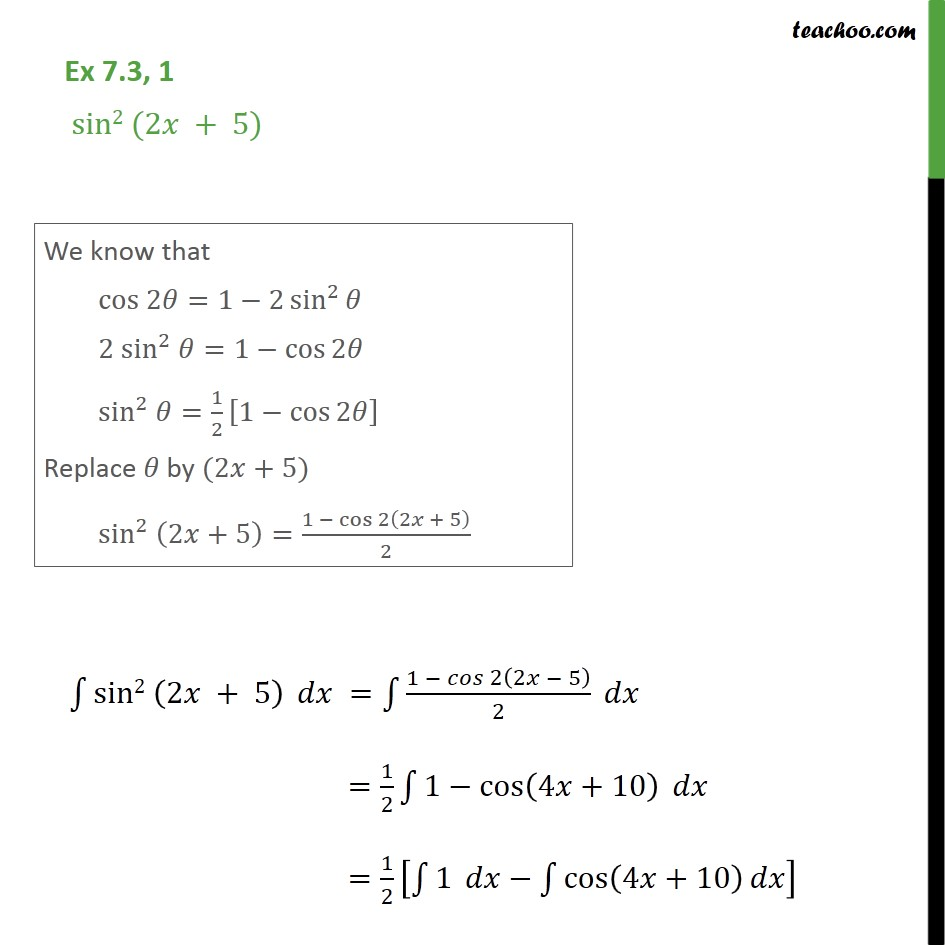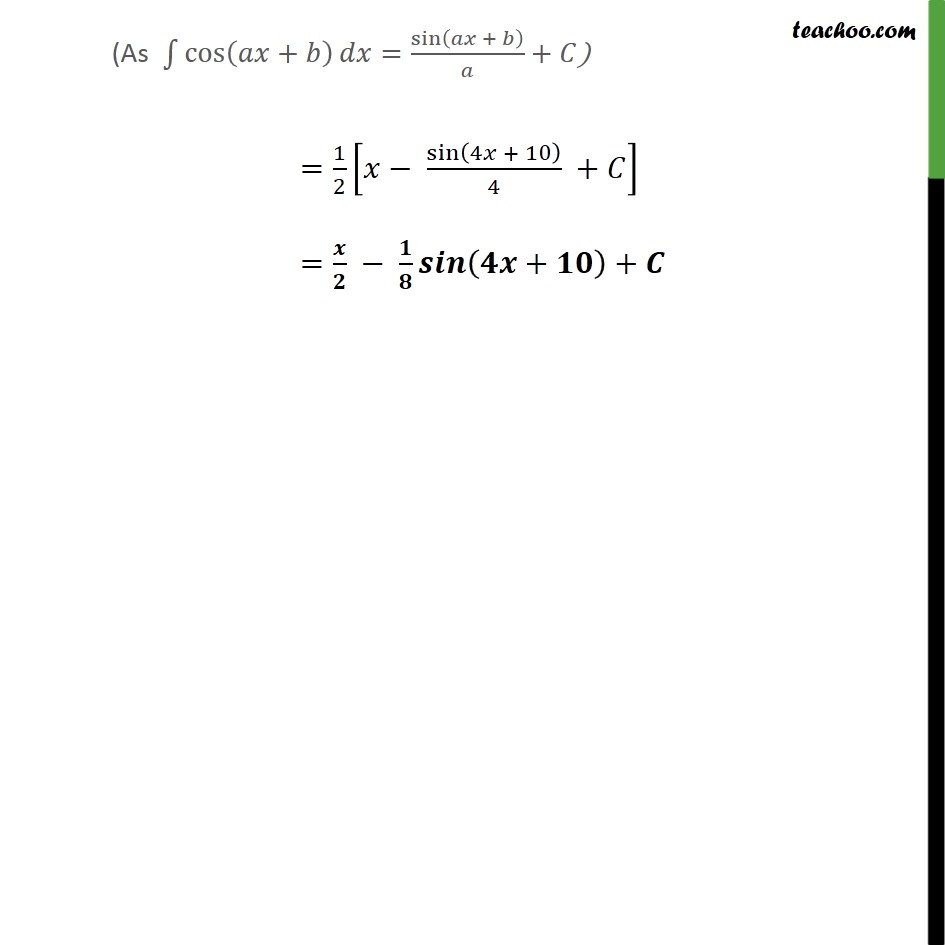1. Chapter 7 Class 12 Integrals
2. Concept wise
3. Integration using trigo identities - 2x formulae

Transcript

Ex 7.3, 1 sin2 (2 + 5) sin2 2 + 5 = 1 2 2 5 2 = 1 2 1 cos 4 +10 = 1 2 1 cos 4 +10 = 1 2 sin 4 + 10 4 + = + +

Integration using trigo identities - 2x formulae